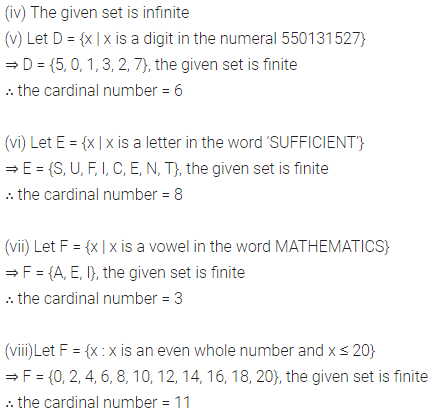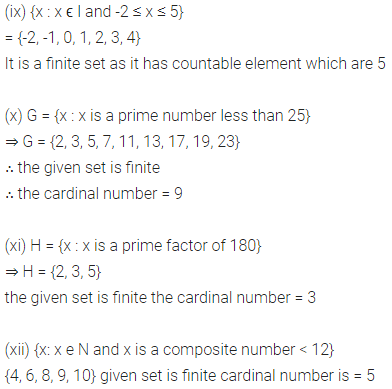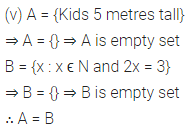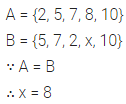# ML Aggarwal Class 6 Solutions for ICSE Maths Chapter 5 Sets Ex 5.2

## ML Aggarwal Class 6 Solutions for ICSE Maths Chapter 5 Sets Ex 5.2

Question 1.
State whether the following sets into empty, finite and infinite sets. In case of (non-empty) finite sets, mention the cardinal number.
(i) {all colours of a rainbow}
(ii) {x | x is a prime number between 7 and 11}
(iii) {multiples of 5}
(iv) {all straight lines drawn in a plane}
(v) {x | x is a digit in the numeral 550131527}
(vi) {x | x is a letter in the word ‘SUFFICIENT’}
(vii) {x | x is a vowel in the word MATHEMATICS}
(viii) {x : x is an even whole number and x ≤ 20}
(ix) {x : x ϵ I and -2 ≤ x ≤ 5}
(x) {x : x is a prime number less than 25}
(xi) {x : x is a prime factor of 180}.
(xii) {x : x ϵ N and x is a composite number < 12}
Solution:Question 2.
State whether the following pairs of sets are equal or not:
(i) A = {2, 4, 6, 8, 10}, B = {even natural numbers}
(ii) A = {3, 5, 7, 9, 11, 13}, B = {odd numbers between 2 and 14}
(iii) A = {PUPPET}, B = {P, U, E, T}
(iv) A = {x | x is a letter in the word SOPHIA}
B = {x | x is a letter in the word MUMTAZ}
(v) A = {kids 5 metres tall}, B = {x : x ϵ N and 2x = 3}.
Solution:Question 3.
Given that A = {2, 5, 7, 8, 10}, B = {5, 7, 2, x, 10} and A = B, write the value of x.
Solution: